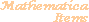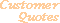Home Older Mathematica Items

Scientific Arts

# Maxwellian Probability Distribution Function

Page 1 | Page 2 | Page 3 | Page 4

## The Distribution Function and its Cumulative

The Maxwellian probability distribution function (PDF) is proportional to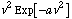where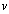is the molecular speed and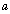is a parameter that depends on the temperature and molecular mass. The normalization factor is given by

``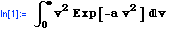``
`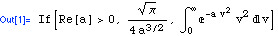`

So a straightforward definition of the Maxwellian PDF is

``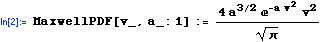``

We have defined MaxwellPDF so that, if it is called with just one argument, a default value of 1 is used for. It is sufficient to consider just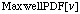since

``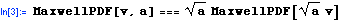``
`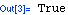`

For molecular dynamicshas the value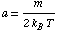where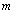is the mass of the molecular species,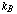is the Boltzmann constant, and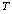is the temperature (in Kelvin). This corresponds to,

``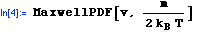``
`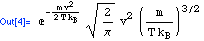`

To calculate random numbers with a probability distribution that conforms to the Maxwellian PDF, we need to compute the cumulative distribution function and invert it.

Here is the cumulative distribution function.

``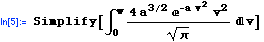``
`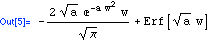`

So we can define the cumulative Maxwellian distribution function via

``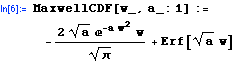``

Similar to the case with MaxwellPDF, we have

``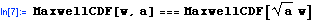``
`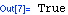`

So we will just work with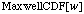to generate random numbers. The result can always be rescaled by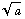.

## Random Numbers

To generate random numbers according to a given probability distribution function, one can use the inverse of the cumulative distribution function acting on random numbers generated from a uniform distribution. In the case of MaxwellCDF there is no closed form inverse.

``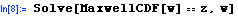``
`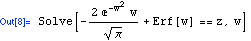`

However, we can build a numerical function that provides us with an inverse. There are many ways to do this. We describe several possibilities in the pages that follow.

### Inverse Series Method with Padé Approximants

[Parts of this example are based on an example given in The Mathematica Journal.]

 For further information on our services send email to info@scientificarts.com . Contents of this web site Copyright © 1999-2011, Scientific Arts, LLC.
 s